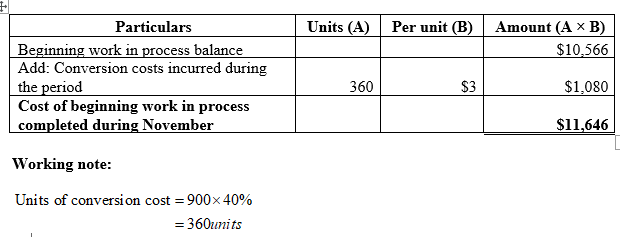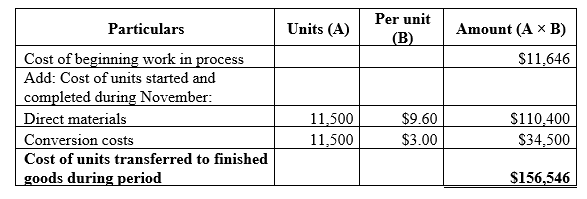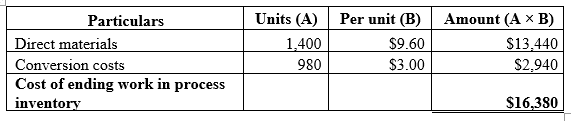# The following information concerns production in the Forging Department for November. All direct materials are placed into the process at the beginning of production, and conversion costs are incurred evenly throughout the process. The beginning inventory consists of \$9,000 of direct materials.ACCOUNT Work in Process—Forging DepartmentACCOUNT NO.DateItemDebitCreditBalanceDebitCreditNov.1Bal., 900 units, 60% completed  10,566   30Direct materials, 12,900 units123,840  134,406   30Direct labor21,650  156,056   30Factory overhead16,870   172,926   30Goods transferred, ? units ? ?   30Bal., 1,400 units, 70% completed  ?  Cost per equivalent units of \$9.60 for Direct Materials and \$3.00 for Conversion Costs.Based on the above data, determine each of the following amounts.If required, round your interim calculations to two decimal places. Round final answers (a-c) to the nearest dollar.a.  Cost of beginning work in process inventory completed in November.\$b.  Cost of units transferred to the next department during November.\$c.  Cost of ending work in process inventory on November 30.\$d.  Costs per equivalent unit of direct materials and conversion included in the November 1 beginning work in process. If required, round your answers to two decimal places.Direct materials cost per equivalent unit\$Conversion cost per equivalent unit\$e.  The November increase or decrease in costs per equivalent unit for direct materials and conversion from the previous month. If required, round your answers to two decimal places. Increase or DecreaseAmountChange in direct materials cost per equivalent unitDecrease  DecreaseIncrease\$Change in conversion cost per equivalent unitIncrease  DecreaseIncrease\$

Question
113 views
1. The following information concerns production in the Forging Department for November. All direct materials are placed into the process at the beginning of production, and conversion costs are incurred evenly throughout the process. The beginning inventory consists of \$9,000 of direct materials.

 ACCOUNT Work in Process—Forging Department ACCOUNT NO. Date Item Debit Credit Balance Debit Credit Nov. 1 Bal., 900 units, 60% completed 10,566 30 Direct materials, 12,900 units 123,840 134,406 30 Direct labor 21,650 156,056 30 Factory overhead 16,870 172,926 30 Goods transferred, ? units ? ? 30 Bal., 1,400 units, 70% completed ?

Cost per equivalent units of \$9.60 for Direct Materials and \$3.00 for Conversion Costs.

Based on the above data, determine each of the following amounts.

If required, round your interim calculations to two decimal places. Round final answers (a-c) to the nearest dollar.

a.  Cost of beginning work in process inventory completed in November.
\$

b.  Cost of units transferred to the next department during November.
\$

c.  Cost of ending work in process inventory on November 30.
\$

d.  Costs per equivalent unit of direct materials and conversion included in the November 1 beginning work in process. If required, round your answers to two decimal places.

 Direct materials cost per equivalent unit \$ Conversion cost per equivalent unit \$

e.  The November increase or decrease in costs per equivalent unit for direct materials and conversion from the previous month. If required, round your answers to two decimal places.

 Increase or Decrease Amount Change in direct materials cost per equivalent unit Decrease    Decrease Increase \$ Change in conversion cost per equivalent unit Increase    Decrease Increase \$

•

check_circle

star
star
star
star
star
1 Rating
Step 1

(a)

Calculate costs of beginning work in process inventory completed in November as shown below:Step 2

(b)

Calculate the cost of units transferred to the next department during November as shown below:Step 3

(c)

Calculate the cost of ending work in process inventory on November 30 as shown below:...

### Want to see the full answer?

See Solution

#### Want to see this answer and more?

Solutions are written by subject experts who are available 24/7. Questions are typically answered within 1 hour.*

See Solution
*Response times may vary by subject and question.
Tagged in